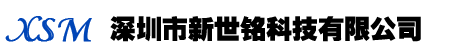行业动态

## 三星贴片电容规格对照表

1 系列编码：

CL=积层陶瓷电容

2 尺寸编码

03=0201(0603) 21=0805(2012) 42=1808(4520)

05=0402(1005) 31=1206(3216) 43=1812(4532)

10=0603(1608) 32=1210(3225) 55=2220(5750)

14=0504(1410) 01=0306(0816) 12=0508(1220)

3 介质：

I类 II类

C=C0G S=S2H L=S2L P=P2H T=T2H

R=R2H U=U2J A=X5R F=Y5V B=X7R X=X6S

4 容量

5 电容的误差：

B0.1pf F=±1pf±1% K=±10%

C=±0.25pf G=±2% M=±20%

D=±0.5pf? J=±5% Z=+80/-20%

6 额定电压：

R=4V O =16V B =50VE = 250V I = 1000V

Q=6.3VA =25V C=100V G = 500VJ = 2000V

P =10VL =35V D =200VH = 630V K= 3000V

7 厚度：

3=0.30毫米 A=0.65毫米 M=1.15毫米 I=2.00毫米 Q=1.25毫米

5=0.50毫米 C=0.85毫米 F=1.25毫米 J=2.50毫米 V=2.50毫米

8=0.80毫米 D=1.00毫米 H=1.60毫米 L=3.20毫米

8 内电极

A=常规产品 钯/银/镍屏蔽/锡 100%

N=常规产品 镍/铜/镍屏蔽/锡 100%

G=常规产品 铜/铜/镍屏蔽/锡 100%

L=低侧面产品 镍/铜/镍屏蔽/锡 100

9 产品编码

A =阵列(2-元素) L =LICC

B =阵列(4-元素)

N =常规

P =自动

C=高频

10 特殊编码

11 包装编码

B=散装

O=纸版箱料带,10英寸料盘

E=压花纸版箱,7英寸料盘

P=散装箱

D=纸版箱料带,13英寸料盘(10000ea)

F=压花纸版箱,13英寸料盘

C=纸版箱料带,7英寸料盘

L=纸版箱料带,3英寸料盘(15,000ea)

S=压花纸版箱,10英寸料盘

 关于我们 IC Product s 新闻资讯 合作品牌 服务客户 联系我们 深圳市新世铭科技有限公司 地址:深圳市龙岗区横岗街道深华街1号林展财富大厦308 电话:86-755-82945939、86-755-83790999 传真:86-755-83298555 电子邮件:Rogerwan@xsmtec.com